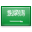# Multivariable calculus

Multivariable calculus (also known as multivariate calculus) is the extension of calculus in one variable to calculus with functions of several variables: the differentiation and integration of functions involving multiple variables, rather than just one.

## Typical operations

### Limits and continuity

A study of limits and continuity in multivariable calculus yields many counter-intuitive results not demonstrated by single-variable functions.:19–22 For example, there are scalar functions of two variables with points in their domain which give different limits when approached along different paths. E.g., the function

${displaystyle f(x,y)={frac {x^{2}y}{x^{4}+y^{2}}}}$approaches zero whenever the point ${displaystyle (0,0)}$is approached along lines through the origin (${displaystyle y=kx}$). However, when the origin is approached along a parabola ${displaystyle y=pm x^{2}}$, the function value has a limit of ${displaystyle pm 0.5}$. Since taking different paths toward the same point yields different limit values, a general limit does not exist there.

Continuity in each argument not being sufficient for multivariate continuity can also be seen from the following example.:17–19 In particular, for a real-valued function with two real-valued parameters, ${displaystyle f(x,y)}$, continuity of ${displaystyle f}$in ${displaystyle x}$for fixed ${displaystyle y}$and continuity of ${displaystyle f}$in ${displaystyle y}$for fixed ${displaystyle x}$does not imply continuity of ${displaystyle f}$.

Consider

${displaystyle f(x,y)={egin{cases}{frac {y}{x}}-y&{ ext{if}}quad 0leq yIt is easy to verify that this function is zero by definition on the boundary and outside of the quadrangle ${displaystyle (0,1) imes (0,1)}$. Furthermore, the functions defined for constant ${displaystyle x}$and ${displaystyle y}$and ${displaystyle 0leq aleq 1}$by

${displaystyle g_{a}(x)=f(x,a)quad }$and ${displaystyle quad h_{a}(y)=f(a,y)quad }$are continuous. Specifically,

${displaystyle g_{0}(x)=f(x,0)=h_{0}(0,y)=f(0,y)=0}$for all x and y.

However, the sequence ${displaystyle fleft({ frac {1}{n}},{ frac {1}{n}} ight)}$(for natural ${displaystyle n}$) converges to ${displaystyle lim _{n o infty }fleft({ frac {1}{n}},{ frac {1}{n}} ight)=1}$, rendering the function as discontinuous at ${displaystyle (0,0)}$. Approaching the origin not along parallels to the ${displaystyle x}$- and ${displaystyle y}$-axis reveals this discontinuity.

### Partial differentiation

The partial derivative generalizes the notion of the derivative to higher dimensions. A partial derivative of a multivariable function is a derivative with respect to one variable with all other variables held constant.:26ff

Partial derivatives may be combined in interesting ways to create more complicated expressions of the derivative. In vector calculus, the del operator (${displaystyle abla }$) is used to define the concepts of gradientdivergence, and curl in terms of partial derivatives. A matrix of partial derivatives, the Jacobian matrix, may be used to represent the derivative of a function between two spaces of arbitrary dimension. The derivative can thus be understood as a linear transformation which directly varies from point to point in the domain of the function.

Differential equations containing partial derivatives are called partial differential equations or PDEs. These equations are generally more difficult to solve than ordinary differential equations, which contain derivatives with respect to only one variable.:654ff

### Multiple integration

The multiple integral expands the concept of the integral to functions of any number of variables. Double and triple integrals may be used to calculate areas and volumes of regions in the plane and in space. Fubini's theorem guarantees that a multiple integral may be evaluated as a repeated integral or iterated integral as long as the integrand is continuous throughout the domain of integration.:367ff

The surface integral and the line integral are used to integrate over curved manifolds such as surfaces and curves.

### Fundamental theorem of calculus in multiple dimensions

In single-variable calculus, the fundamental theorem of calculus establishes a link between the derivative and the integral. The link between the derivative and the integral in multivariable calculus is embodied by the integral theorems of vector calculus::543ff

In a more advanced study of multivariable calculus, it is seen that these four theorems are specific incarnations of a more general theorem, the generalized Stokes' theorem, which applies to the integration of differential forms over manifolds.

## Applications and uses

Techniques of multivariable calculus are used to study many objects of interest in the material world. In particular,

Type of functions Applicable techniques
Curves${displaystyle f:mathbb {R} o mathbb {R} ^{n}}$for ${displaystyle n>1}$Lengths of curves, line integrals, and curvature.
Surfaces${displaystyle f:mathbb {R} ^{2} o mathbb {R} ^{n}}$for ${displaystyle n>2}$Areas of surfaces, surface integralsflux through surfaces, and curvature.
Scalar fields${displaystyle f:mathbb {R} ^{n} o mathbb {R} }$Maxima and minima, Lagrange multipliersdirectional derivativeslevel sets.
Vector fields${displaystyle f:mathbb {R} ^{m} o mathbb {R} ^{n}}$Any of the operations of vector calculus including gradientdivergence, and curl.

Multivariable calculus can be applied to analyze deterministic systems that have multiple degrees of freedom. Functions with independent variables corresponding to each of the degrees of freedom are often used to model these systems, and multivariable calculus provides tools for characterizing the system dynamics.

Multivariate calculus is used in the optimal control of continuous time dynamic systems. It is used in regression analysis to derive formulas for estimating relationships among various sets of empirical data.

Multivariable calculus is used in many fields of natural and social science and engineering to model and study high-dimensional systems that exhibit deterministic behavior. In economics, for example, consumer choice over a variety of goods, and producer choice over various inputs to use and outputs to produce, are modeled with multivariate calculus. Quantitative analysts in finance also often use multivariate calculus to predict future trends in the stock market.

Non-deterministic, or stochastic systems can be studied using a different kind of mathematics, such as stochastic calculus.

### أهلاً ومرحباً بكم## قسم الرياضيات### التوقيت والتقويم

```_time.start(["thetimenow"]);
```

```
```

```

```

### محرك بحث جوجل```

```

### للتواصل1. الهاتف : 0164044771

تحويلة: 4771

(QR Code)

mailto:mm.mousa@mu.edu.sa### إعلانات1- الاختبار الفصلى الثانى لمقرر التحليل العددى (يوم الاحد الموافق 3 / 7/ 1440 هـ)

2- الاختبار الفصلى الثانى لمقررحساب المتجهات (يوم الثلاثاء الموافق 5 / 7 / 1440 هـ)

### الساعات المكتبيةالأثنين: 10 - 12

الثلاثاء: 8 - 10

الأربعاء: 8 - 10

### بعض الخدمات الإلكترونية لعمادة تقنية المعلومات### آلة حاسبة

```

```

### التقويم الجامعى### بعض الجوائز والتكريمات### إحصائية الموقع

عدد الصفحات: 258

البحوث والمحاضرات: 155

الزيارات: 101561